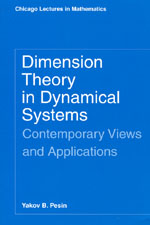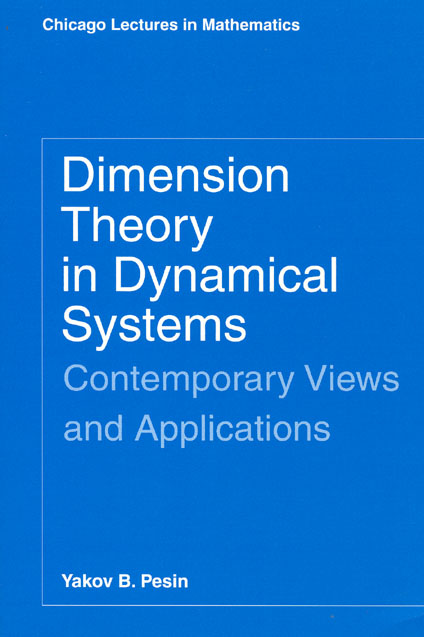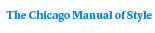# Dimension Theory in Dynamical Systems

## Contemporary Views and Applications

Yakov B. Pesin311 pages | 6 x 9 | © 1997
Paper \$37.00 ISBN: 9780226662220 Published December 1997
E-book \$10.00 to \$37.00 About E-books ISBN: 9780226662237 Published April 2008
The principles of symmetry and self-similarity structure nature’s most beautiful creations. For example, they are expressed in fractals, famous for their beautiful but complicated geometric structure, which is the subject of study in dimension theory. And in dynamics the presence of invariant fractals often results in unstable "turbulent-like" motions and is associated with "chaotic" behavior.

In this book, Yakov Pesin introduces a new area of research that has recently appeared in the interface between dimension theory and the theory of dynamical systems. Focusing on invariant fractals and their influence on stochastic properties of systems, Pesin provides a comprehensive and systematic treatment of modern dimension theory in dynamical systems, summarizes the current state of research, and describes the most important accomplishments of this field.

Pesin’s synthesis of these subjects of broad current research interest will be appreciated both by advanced mathematicians and by a wide range of scientists who depend upon mathematical modeling of dynamical processes.
Table of Contents• Contents
Contents
Preface
Introduction
Part I: Carathéodory Dimension Characteristics
Chapter 1. General Carathéodory Construction
1. Carathéodory Dimension of Sets
2. Carathéodory Capacity of Sets
3. Carathéodory Dimension and Capacity of Measures
4. Coincidence of Carathéodory Dimension and Carathéodory Capacity of Measures
5. Lower and Upper Bounds for Carathéodory Dimension of Sets; Carathéodory Dimension Spectrum
Chapter 2. C-Structures Associated with Metrics: Hausdorff Dimension and Box Dimension
6. Hausdorff Dimension and Box Dimension of Sets
7. Hausdorff Dimension and Box Dimension of Measures; Pointwise Dimension; Mass Distribution Principle
Chapter 3. C-Structures Associated with Metrics and Measures: Dimension Spectra
8. q-Dimension and q-Box Dimension of Sets
9. q-Dimension and q-Box Dimension of Measures
Appendix I: Hausdorff (Box) Dimension and Q-(Box) Dimension of Sets and Measures in General Metric Spaces
Chapter 4. C-Structures Associated with Dynamical Systems: Thermodynamic Formalism
10. A Modification of the General Carathéodory Construction
11. Dimensional Definition of Topological Pressure; Topological and Measure-Theoretic Entropies
Appendix II: Variational Principle for Topological Pressure; Symbolic Dynamical Systems; Bowen’s Equation
Appendix III: An Example of Carathéodory Structure Generated by Dynamical Systems
Part II: Applications to Dimension Theory and Dynamical Systems
Chapter 5. Dimension of Cantor-like Sets and Symbolic Dynamics
13. Moran-like Geometric Constructions with Stationary (Constant) Ratio Coefficients
14. Regular Geometric Constructions
15. Moran-like Geometric Constructions with Non-stationary Ratio Coefficients
16. Geometric Constructions with Rectangles; Non-coincidence of Box Dimension and Hausdorff Dimension of Sets
Chapter 6. Multifractal Formalism
17. Correlation Dimension
18. Dimension Spectra: Hentschel-Procaccia, Rényi, and f(alpha)-Spectra; Information Dimension
19. Multifractal Analysis of Gibbs Measures on Limit Sets of Geometric Constructions
Chapter 7. Dimension of Sets and Measures Invariant under Hyperbolic Systems
20. Hausdorff Dimension and Box Dimension of Conformal Repellers for Smooth Expanding Maps
21. Multifractal Analysis of Gibbs Measures for Smooth Conformal Expanding Maps
22. Hausdorff Dimension and Box Dimension of Basic Sets for Axiom A Diffeomrophisms
23. Hausdorff Dimension of Horseshoes and Solenoids
24. Multifractal Analysis of Equilibrium Measures on Basic Sets of Axiom A Diffeomorphisms
Appendix IV: A General Concept of Multifractal Spectra; Multifractal Rigidity
Chapter 8. Relations between Dimension, Entropy, and Lyapunov Exponents
25. Existence and Non-existence of Pointwise Dimension for Invariant Measures
26. Dimension of Measures with Non-zero Lyapunov Exponents; The Eckmann-Ruelle Conjecture
Appendix V: Some Useful Facts
Bibliography
Index